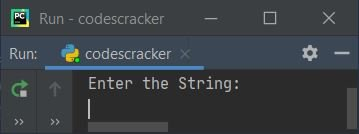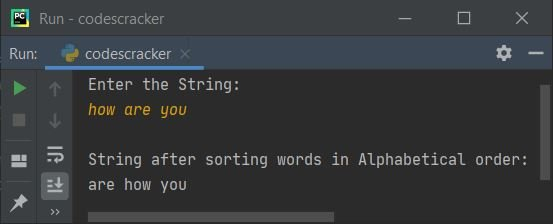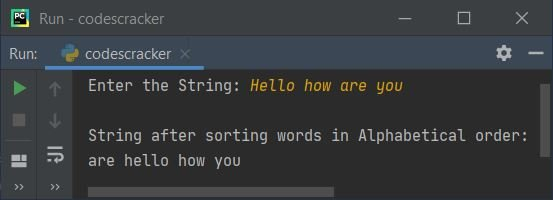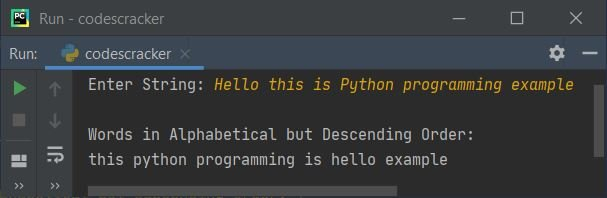# Python Program to Sort Words in Alphabetical Order

This article deals with some programs in Python that sorts all the words in a given string by user at run-time, in alphabetical order. Here are the list of programs covered in this article:

• Sort words in alphabetical order
• Sort words in alphabetical but in descending order

## Sort Words in Alphabetical Order

The question is, write a Python program that receives a string as input from user and sorts all the words of given string in alphabetical order. Here is its answer:

```print("Enter the String: ")
str = input()

wrds = str.split()
wrds.sort()

sortedwrds = ""
for wrd in wrds:
sortedwrds = sortedwrds + wrd + " "

print(sortedwrds)```

This program produces initially the output like shown in the snapshot given below:Now provide the input say how are you and press `ENTER` key to sort and print the same string but all of its words gets sorted in alphabetical order like shown in the snapshot given below:Question - What if user enters a string that contains some words in capital letter.

To avoid any problem like above question, we've to modify the previous program. That is, we must have to convert all the word in given string by user, in lowercase before sorting it. So let's create another program for it.

#### Modified Version of Previous Program

This program splits the given string into words using split() method. The using for loop, each word gets converted into lowercase one by one. After doing this task, I've done the task of sorting all the words in alphabetical order like shown in the program given below:

```print("Enter the String: ", end="")
str = input()

wrds = str.split()

for i in range(len(wrds)):
wrds[i] = wrds[i].lower()

wrds.sort()

sortedwrds = ""
for wrd in wrds:
sortedwrds = sortedwrds + wrd + " "

print("\nString after sorting words in Alphabetical order:")
print(sortedwrds)```

Here is its sample run with user input, Hello how are you:## Sort Words in Alphabetical but in Descending Order

To sort list of words in a given string in alphabetical order, but in descending order, just add the following code:

`reverse=True`

inside the bracket of

`wrds.sort()`

Here is the complete version of the program:

```print("Enter String: ", end="")
str = input()

wrds = str.split()

for i in range(len(wrds)):
wrds[i] = wrds[i].lower()

wrds.sort(reverse=True)

sortedwrds = ""
for wrd in wrds:
sortedwrds = sortedwrds + wrd + " "

print("\nWords in Alphabetical but Descending Order:")
print(sortedwrds)```

Here is its sample run with user input, Hello this is Python programming example:Python Online Test

« Previous Program Next Program »